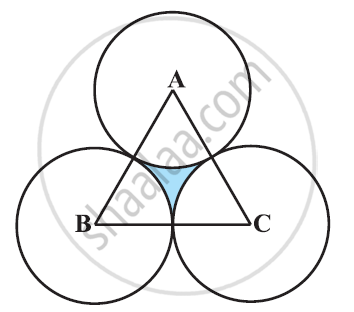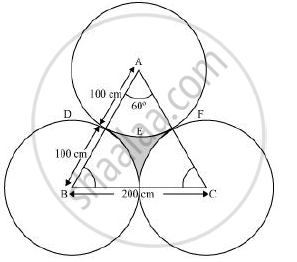Share

# The area of an equilateral triangle ABC is 17320.5 cm2. With each vertex of the triangle as centre, a circle is drawn with radius equal to half the length of the side of the triangle (See the given figure). Find the area of shaded region. - CBSE Class 10 - Mathematics

ConceptAreas of Combinations of Plane Figures

#### Question

The area of an equilateral triangle ABC is 17320.5 cm2. With each vertex of the triangle as centre, a circle is drawn with radius equal to half the length of the side of the triangle (See the given figure). Find the area of shaded region. [Use π = 3.14 and sqrt3 = 1.73205]#### Solution

Let the side of the equilateral triangle be a.

Area of equilateral triangle = 17320.5 cm2

sqrt3/4(s)^2 = 17320.5

1.7320/4a^2 = 17320.5

a2 = 4 x 10000

a = 200 cmEach sector is of measure 60°.

Area of sector ADEF = 60^@/360^@ xx pixxr^2

=1/6xxpixx(100)^2

=(3.14xx10000)/6

= 15700/3 cm^2

Area of shaded region = Area of equilateral triangle − 3 × Area of each sector

= 17320.5 - 3xx 15700/3

= 17320.5-15700 = 1620.5 cm2

Is there an error in this question or solution?

#### APPEARS IN

NCERT Solution for Mathematics Textbook for Class 10 (2019 to Current)
Chapter 12: Areas Related to Circles
Ex. 12.30 | Q: 10 | Page no. 236
Solution The area of an equilateral triangle ABC is 17320.5 cm2. With each vertex of the triangle as centre, a circle is drawn with radius equal to half the length of the side of the triangle (See the given figure). Find the area of shaded region. Concept: Areas of Combinations of Plane Figures.
S